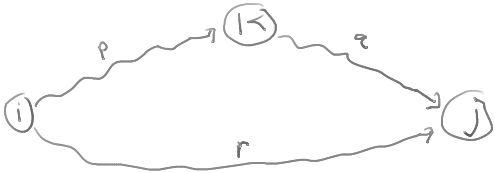# ShortestPathsByDP

ClassS04CS141 | recent changes | Preferences

### Shortest Paths by Dynamic Programming

Recall that if all edge weights are non-negative, Dijkstra's algorithm computes the shortest path distances from a given source vertex S to all other vertices.

All of the algorithms considered here will work even if there are negative-weight edges in the graph (as long as there are no negative-cost cycles).

#### 1. Shortest paths in a directed acyclic graph

This algorithm computes shortest path distances from a given source vertex S to all other vertices in the digraph, assuming the digraph is acyclic.

Order the vertices v1,v2,...,vn in topological order.

Define

D[i] = length of shortest path from S to vi.

Since all paths in the graph go forward in the topological ordering, the following recurrence holds:

D[i] = min { D[j] + WT(j,i) : (j,i) ∈ E, j < i }

Base case:

D[i0] = 0 where S = vi0
D[i] = ∞ where i < i0

Algorithm:

``` 1. For i = 1,2,...,i0-1
2.   D[i] = ∞
3. D[i0] = 0
4. For i =  i0+1, i0+2,...,n
5.   D[i] = min { D[j] + WT(j,i) : (j,i) ∈ E, j < i }
6. Return D
```

This algorithm runs in O(n+m) time, since each edge is considered once in line 5.

#### 2. Bellman-Ford Algorithm

Here is another algorithm that computes shortest path distances from a given source vertex S to all other vertices. This one is based on dynamic programming and works even if the graph has negative-weight edges, as long as the graph has no negative-weight cycles.

Order the vertices v1,v2,...,vn in some arbitrary way. Define

D[i,k] = length of shortest path from S to i using a path of length at most k.

Here are the base cases for the recurrence relation:

D[i,0] = 0 if i=S, otherwise D[i,0] = ∞.

Here is the recurrence relation. For k > 0,

D[i,k] = min { D[i,k-1], min { D[j, k-1] + WT(j,i) : (j,i) ∈ E } }.

This gives the following algorithm:

``` 1. for each vertex i, set D[i] = ∞
2. D[S] = 0
3. for k = 1,2,...,n-1
4.    D[i] = min { D[i], min { D[j] + WT(j,i) : (j,i) ∈ E } }.
5. return D.
```

This algorithm runs in O(nm) time, since line 4 can be implemented in O(m) time.

How can we modify this algorithm to detect if the graph has a negative-weight cycle?

#### 3. Floyd-Warshall Algorithm

The next algorithm computes shortest path distances between all pairs of vertices. It also works as long as there are no negative-weight cycles in the graph.

The algorithm is based on TransitiveClosureByDP algorithm.

Order the vertices v1,v2,...,vn in some arbitrary way.

Define D[i,j,k] to be the length of the shortest path from i to j that goes through only the first k vertices v1,...,vk. (That is, i and j need not be in the set of k vertices, but all the other vertices on the path must be.)

Claim: Assume the graph has no negative-weight cycles. Then, for k > 0, D[i,j,k] = min { D[i,j,k-1], D[i,k,k-1] + D[k,j,k-1] }

To see this, consider the diagram:A path is simple if it contains no cycles. If the graph has no negative-weight cycles, then between any two vertices there is always a shortest path that is simple (as long as there is some path).

Any simple path from i to j going only through vertices in the set {1,2,...,k} is of one of the following two forms:

1. The path goes from i to j using only vertices in the set {1,2,...,k-1}.
2. The path goes from i to k using only vertices in the set {1,2,...,k-1} and then goes from k to j using only vertices in the set {1,2,...,k-1}.

The base case for our recurrence relation is

D[i,j,0] = WT(i,j) if (i,j) is an edge, otherwise D[i,j,0] = ∞.

This recurrence leads to the following algorithm:

```  1. Initialize D[i,j] = ∞ for all i,j.
2. Set D[i,j] = WT(i,j) for each edge (i,j) in E.
3. For k = 1,2,...,n
4.   For i = 1,2,...,n
5.     For j = 1,2,...,n
6.        D[i,j] = min { D[i,j],  D[i,k] + D[k,j] }
7. Return D.
```

The algorithm runs in O(n3) time.

Exercise: how do we detect it if the graph does have a negative cycle? (In this case, shortest paths are not well defined, because by going around the cycle repeatedly, we get paths with arbitrarily negative weight.)

### References

ClassS04CS141 | recent changes | Preferences